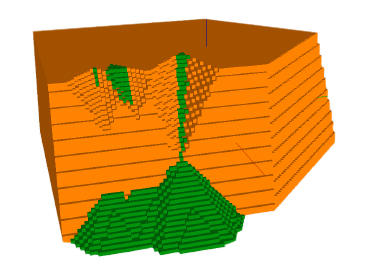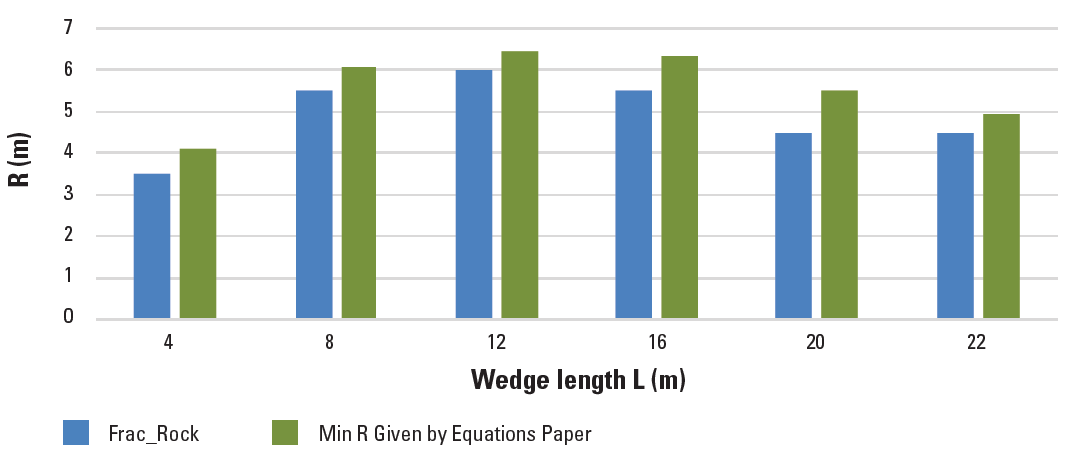# Comparison of Methods for Estimating Berm Widths

SRK News | Issue 54: Rock Engineering and Slope StabilityFigure 2: Interaction of multiple wedgesFor the design of a pit wall, several combinations of bench height, bench face angle and berm width may be used to satisfy stability criteria. The bench face angle and the berm width must be chosen to fit the bench stack angle. The berm must also be wide enough to contain the volume of wedge material from the bench faces above, in the event of failure.

In 2006, Gibson et al proposed two equations for calculating the required spill berm width from the wedge failure volumes, which depend on the geometry of the failed material on the berm (conical vs pyramid shape).

This article compares the berm width obtained using the equations developed by Gibson et al and the results obtained using Frac_Rock, a computer program developed by SRK that uses a discrete fracture network to model the wedge generation and stability.

Based on the results, it seems that modelling the overspill numerically rather than deterministically gives a more optimal answer, even though the equations proposed in 2006 are still valid for preliminary assessment.

Figure 1 below shows the comparison of berm required to contain failed material calculated using the equations and the Frac_Rock model, the results indicate the equation may be a conservative way to assess berm width. The benefit of using a program like Frac_Rock is that the same calculation can be made for multiple interacting wedges like the example shown in Figure 2 above left.

A range of wedges with different sizes were modelled using Frac_Rock and the volume of failed material was calculated and compared with the volumes calculated using the equations. Figure 3a above shows one of the models and Figure 3b shows the location of the failed material.

Figure 1: Comparison of berm width required calculated with equations and Frac_Rock modelWilliam Gibson: wgibson@srk.com.au
Justine Paul: jpaul@srk.com.au

Reference: Gibson, W, de Bruyn, I A and Walker, D J H, 2006. Considerations in the optimisation of bench face angle and berm width geometries for open pit mines, in Proceedings of The South African Institute of Mining and Metallurgy International Symposium on Stability of Rock Slopes, Symposium Series S44 Stability of rock slopes in open pit mining and civil engineering situations, pp. 557 – 579.